# How to Extract Time From A Date And Time in Excel

This post will guide you how to extract time portion from a date and time in Excel. How do I remove date on Date/Time Stamp with a formula in Excel. How to remove date from a date and time value with find and replace feature in Excel 2013/2016.

Assuming that you have a list of data in range B1:B4 in which contain date and time stamps. You want to pull out the time portion only from the given cells in Excel. This post will show you two methods to remote the date from a cell with date and time in it.

## Method1: Extract Time from a Date and Time Using Formula

You can remove the date portion from a date and time stamps by using the TIME function, HOUR function, MINUTE function, and SECOND function. Just see the example below, removing the date portion from those values in range B1:B4 using the following formula:

`=TIME(HOUR(B1),MINUTE(B1),SECOND(B1))`

Type this formula into a blank cell and press Enter key on the keyboard, and drag the AutoFill Handle down to other cells to apply this formula.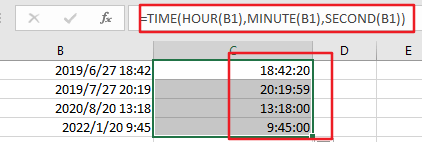Let’s see how this formula works:

The HOUR, MINUTE and SECOND functions are used to extract values for the Hour, Minute and Second portions. Then the TIME function will format those values as a Time stamp.

You can also use another formula based on the INT function to achieve the same result. Like this:

`=B1-INT(B1)`

Type this formula into a blank cell and press Enter key, then drag the AutoFill handle over to the other cells that you want to apply the same formula. The serial number of time portion would be extracted. Then you need to reformat those cells to a time format, it will allow the fraction to show as a normal Time value.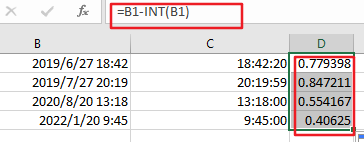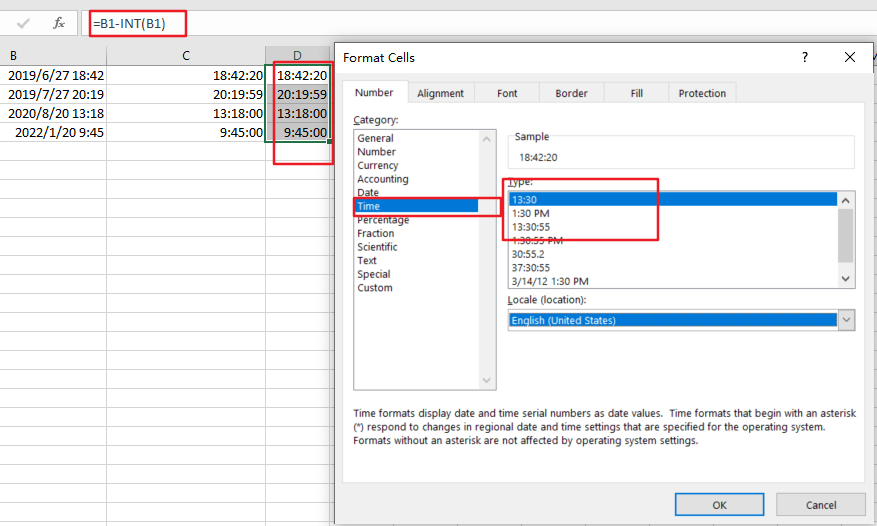## Method2: Extract Time from a Date and Time Using Find and Replace

You can also use Find and Replace feature to extract time portion from a date and time stamp in Excel. Just do the following steps:

Step1: select the range of cells that contain date and time values.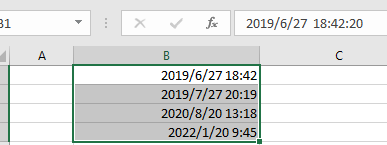Step2: right click on the selected cells, and choose Format Cells from the dropdown menu list. And the Format Cells dialog will open.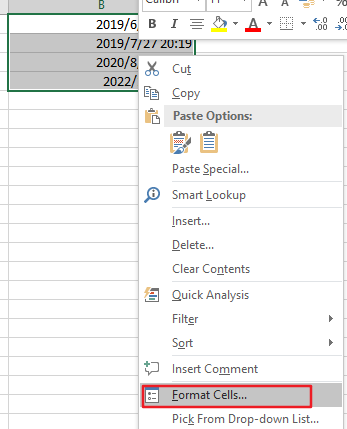Step3: select General category under the Category drop list, it will change the normal date and time forma to general format. click Ok button to return to the current worksheet.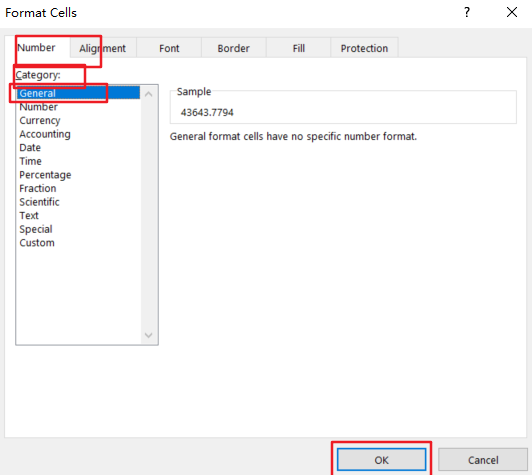Step4: go to Home tab in the Excel Ribbon, click Find & Select command under Editing group, and choose Replace from the dropdown list. And the Find and Replace dialog will open.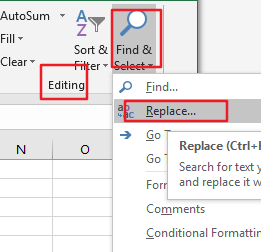Step5: enter the pattern *. Into the Find what text box, and enter the another pattern . into the Replace with text box in the Find and Replace dialog box.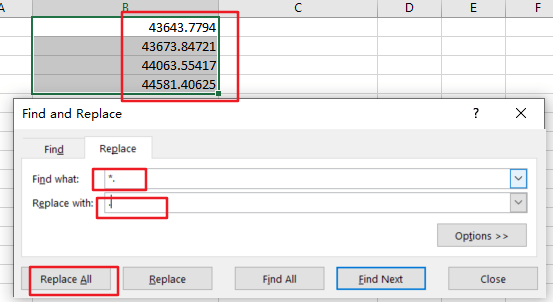Step6: click Replace All button, click close button to back to the current worksheet. You would get the serial number of the time portion for all the selected date and time values.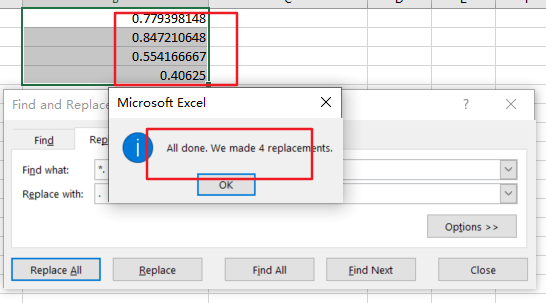Step7: keep those values selected, and right click on it, and select Format cells, and choose Time category under the Category drop list. And select one type in the Type list box, click Ok button.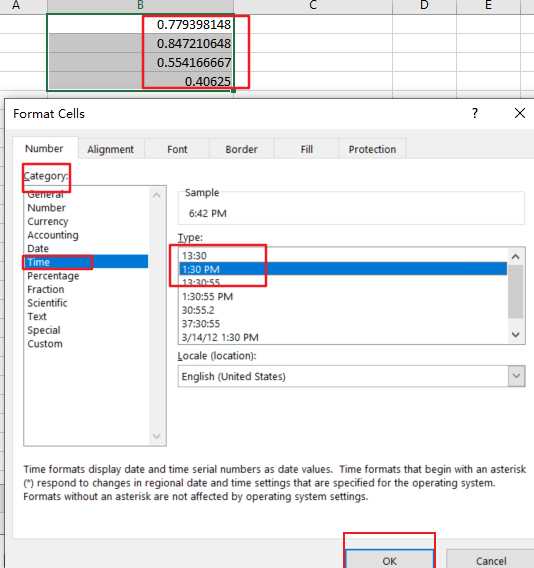Step8: you would see that all time portions have been extracted.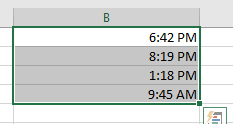### Related Functions

• Excel TIME function
The Excel TIME function returns a date in serial number format for a particular time (the hour, minute and second).The syntax of the TIME function is as below:= TIME(hour, minute, second)…
• Excel HOUR Function
The Excel HOUR function returns the hour of a time value. Or returns an integer value that represent the hour component of a given time. The syntax of the HOUR function is as below:=HOUR (serial_number) …
• Excel MINUTE Function
The Excel MINUTE function returns the minutes of a time value. And the minutes is an integer number from 0 to 59. The syntax of the MINUTE function is as below:=MINUTE (serial_number)…
• Excel SECOND Function
The Excel SECOND function returns the seconds of a time value. Or returns an integer value that represent the second component of a given excel time. And the return value is between 0-59.The syntax of the SECOND function is as below:=SECOND (serial_number)…